## Direction Sense Test Questions and Answers Part-3

1. The front door of Kiran’s house is towards the south. From the backside of her house she walks 50 metres straight then turns towards the left and walks 100 metres and after that turns right and stops after walking 100 metres. Now Kiran is facing which direction?
a) East
b) South
c) West
d) North

Explanation: Since her house faces towards the south, when she starts from the back side of her house she would go 50 metres north. After this when she turns left, she would be going west and when she again turns right she would be facing the north again. Hence, Option (d) is the correct answer.

2. A boy starts walking straight towards the north and walks 10 feet, then he turns to his left and walks 5 feet, then he turns to his left and walks another 5 feet, then again he turns to his left and walks 10 feet and then he turns to his right and walks 2 feet. How far is he now from his starting point?
a) 5 feet
b) 2 feet
c) $\sqrt{34}$ feet
d) 7 feet

Explanation:From the figure, he starts at A goes through B C D E F and his final distance from A is given by the distance A = $\sqrt{(5)^{2} + (3)^{2}} = \sqrt{34}$
Hence, Option (c) is the correct answer

3. A policeman goes 20 km east and then turning to the south he goes 30 km and then again turns to his left and goes 10 km. How far is he from his starting point?
a) 30 km
b) 20 km
c) 10 km
d) 40 km

Explanation: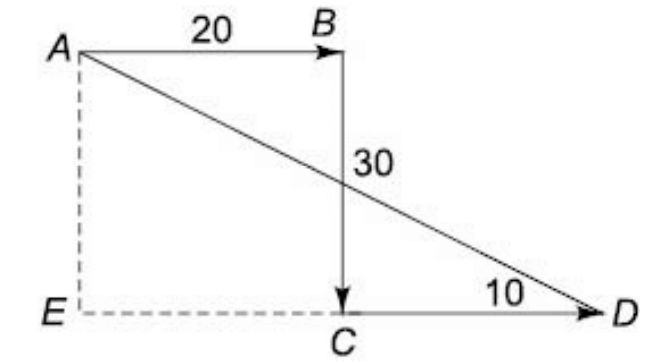Distance AD = $\sqrt{1800} = 30\sqrt{2}$
Hence, Option (c) is the correct answer.

4. I walk 1 km to my east then I turn to the south and walk for 5 km. Next, I turn east and walk 2 km. After this I turn to the north and walk for 9 km. How far am I from my starting point?
a) 5 km
b) 4 km
c) 6 km
d) Can’t be determined

Explanation: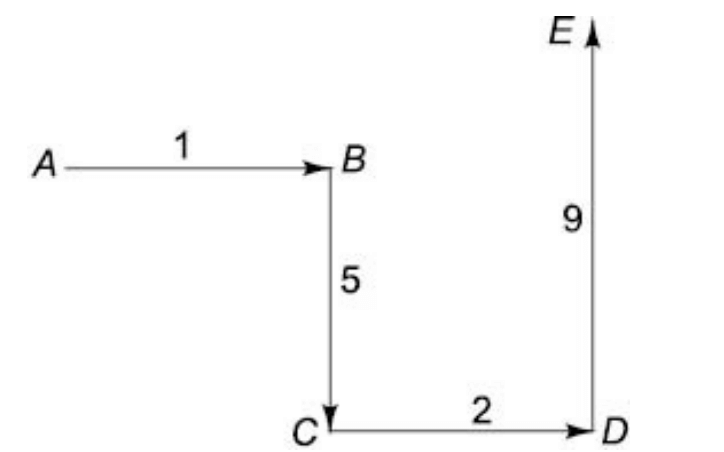d(AE) = $\sqrt{(3)^{2} + (4)^{2}}$   = 5 km
Hence, Option (a) is the correct answer

5. A man walks 5 metres straight and then 10 meters to the right. After this he continuously turns left and every time walks 10, 5 and 10 meters respectively. How far is he now from his starting point?
a) $5\sqrt{3}$ metres
b) $3\sqrt{5}$ metres
c) $5\sqrt{2}$ metres
d) 10 metres

Explanation: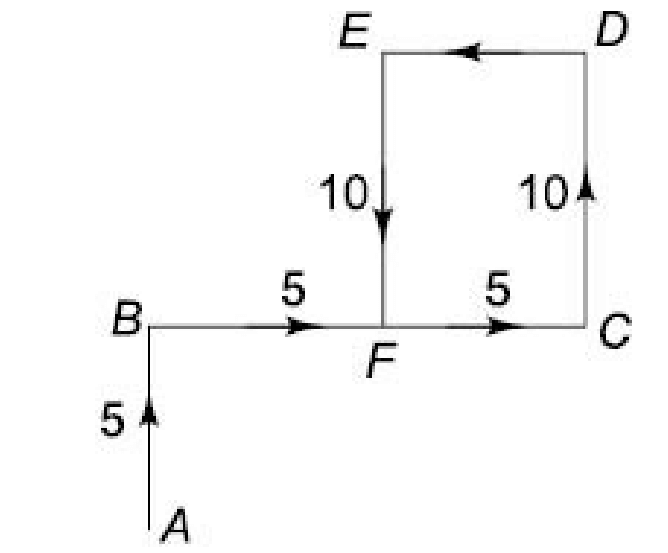If A is the starting point:
The required distance is seen on AF = $\sqrt{50} = 5\sqrt{2}$
Hence, Option (c) is the correct answer.

6. Karan’s house is south facing. From the front door of his house Karan started walking and after walking 20 metres straight he turned to his left and walked 50 metres. After this he turned to his right and walked 80 metres. Find the distance between the point from where he finished his walk and the door of his house.
a) $50\sqrt{5}$  metres
b) $5\sqrt{50}$  metres
c) 100 metres
d) 120 metres

Explanation: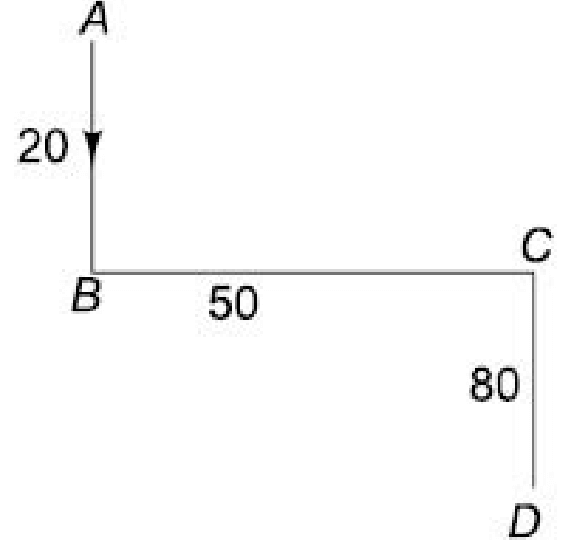d/AD = $\sqrt{50^{2} + 100^{2}}$
$\sqrt{12500} = 50\sqrt{5}$     metres
Hence, Option (a) is the correct answer.

7. A man started walking towards north, he walks 10 metres and then turns to his right, walks 5 metres and turns to his right again and walks 10 metres and turns to his left, walks 7 metres and then turns to his right. At this point in which direction is he facing?
a) East
b) South
c) Southeast
d) Southwest

Explanation: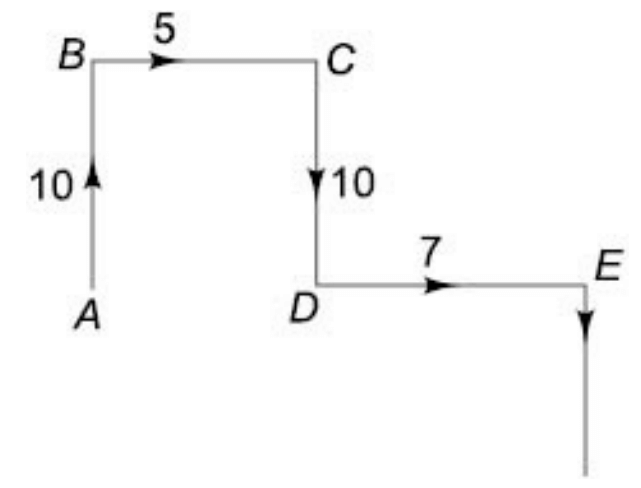Starting from A he would be at E and facing South. Hence, Option (b) is the correct answer

8. If Southeast becomes east and Northwest becomes west and all the other directions are changed in the same direction. Then what will be the direction for north?
a) Northwest
b) Southeast
c) Southwest
d) Northeast

Explanation:From this figure it is clear that there has to be a 45° counter clockwise rotation. Hence, Northwould become northwest. Hence, Option (a) is the correct answer

9.If Northwest becomes south and southwest becomes east and all the other directions change in the similar manner, then what will be the direction for north?
a) Southeast
b) Northeast
c) North
d) None of these